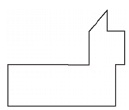# User Forum

Subject :IMO    Class : Class 4

Which set of polygons can be used to compose the following shape?(a) One square and two rectangles
(b) One rectangle, one square, and one triangle
(c) One rectangle, one triangle, and one trapezium
(d) One trapezium, one rectangle, and one square